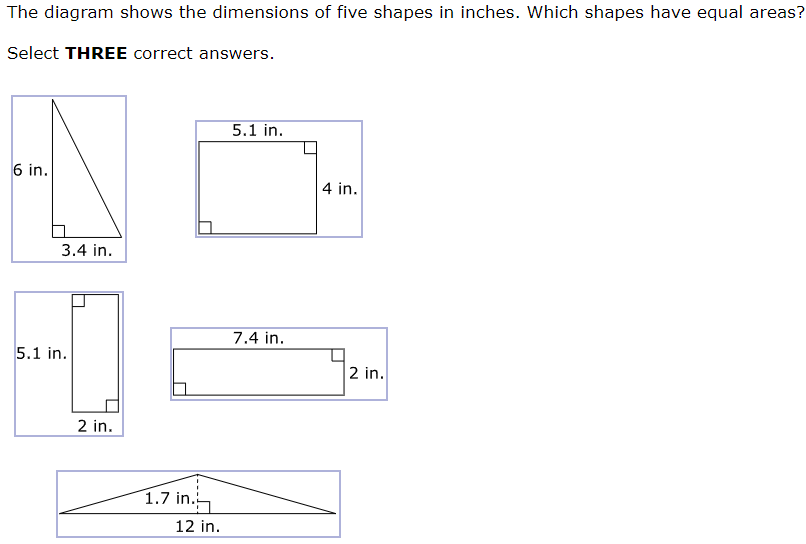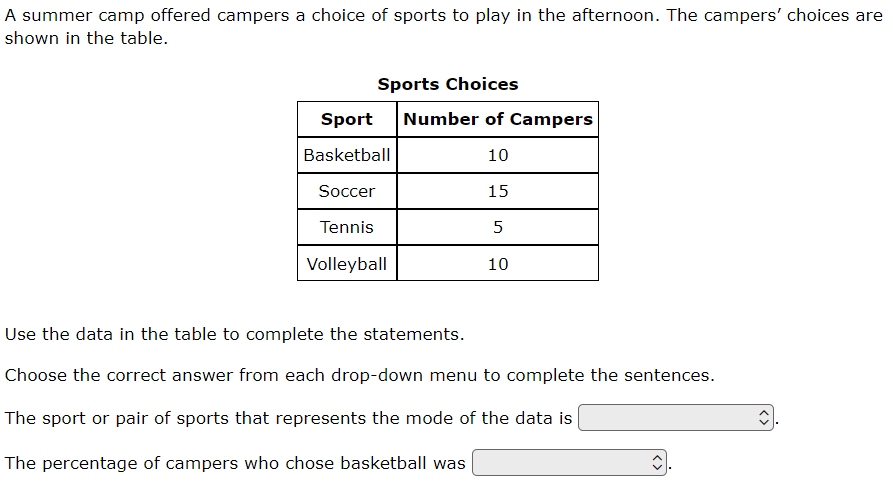top of page
Search

# Lessons learned from the 2023 6th Grade Math STAAR

The 2023 6th Grade Math STAAR introduced statewide online testing and several new item types. Using a modified version of the statewide item analysis report, I examined the readiness standards that had less than 50% mastery. Each standard has both an analysis of the items themselves to infer what made them so difficult and instructional implications for educators to ensure a more successful 2024 STAAR test.

 Standard # of items % mastery 6.8D 1 15 6.12D 1 20 6.11A 2 30.5 6.4B 2 31 6.5B 2 31.5 6.7A 1 33 6.6C 1 38 6.7D 1 38 6.3D 2 44.5 6.3E 2 48 6.4G 2 49.5

Access the slide deck here.

### 6.8D - 15% overall mastery

determine solutions for problems involving the area of rectangles, parallelograms, trapezoids, and triangles and volume of right rectangular prisms where dimensions are positive rational numbers

#25 - 15% full credit, 50% partial credit, 34% no credit###### Analysis
• Multiselect took away certainty of multiple choice

• Correct answers included both triangles and a rectangle

• Students had to use two different formulas for area

###### Instructional Implications
• Practice finding area of multiple shapes (e.g., rectangle, square, parallelogram, triangle, trapezoid) that result in same answer

• Have students manipulate shape dimensions to reach a target area (e.g., What could you change the width/height to in order to make the area 15 square cm?)

### 6.12D - 20% overall mastery

summarize categorical data with numerical and graphical summaries, including the mode, the percent of values in each category (relative frequency table), and the percent bar graph, and use these summaries to describe the data distribution

#5 - 20% full credit, 50% partial credit, 30% no credit###### Analysis
• Students had to know definition of mode and apply it

• Finding percentage of campers who chose basketball required students to first find the total

• Inline choice required accessing the data multiple times for different purposes

###### Instructional Implications
• Find mode, median, and range of ALL data sets

• Practice percentage of the total for single selection or multiple selections (e.g., basketball and soccer)

Watch the full walkthrough of all 36 items on the 2023 6th Grade STAAR below.

### 6.11A - 30.5% overall mastery

graph points in all four quadrants using ordered pairs of rational numbers

#14 - 23% correct#22 - 38% correct###### Analysis
• Graphing item type required placing point on x = -0.5 (scale factor of 1)

• Point Z on #22 was not plotted

• Points were mixed numbers (not integers) distant from given point

• Answer distribution on #22 suggests guessing

###### Instructional Implications
• Utilize mnemonic to memorize 4 quadrants

• Practice plotting half-points in various quadrants

• Find vertical and horizontal distance from a given point in a variety of directions

### 6.4B - 31% overall mastery

apply qualitative and quantitative reasoning to solve prediction and comparison of real-world problems involving ratios and rates

#13 - 35% correct#32 - 27% full credit, 26% partial credit, 47% no credit###### Analysis
• Most chosen response for #13 (B - 38%) didn’t use a ratio, divided 63 by 3

• Correct ratio for #13 used hidden number for total (7)

• Drag and drop for #32 eliminated assurance of multiple choice

• Ratio and table for #32 had no tricks (e.g, ratio of 2:3 set up on table exactly)

###### Instructional Implications
• Have students solve problems involving finding a part if given a ratio and the whole (like #13) and finding a part if given the ratio (like #32)

• Use tables (like #32) so students find multiple missing parts, not just one

### 6.5B - 31.5% overall mastery

solve real-world problems to find the whole given a part and the percent, to find the part given the whole and the percent, and to find the percent given the part and the whole, including the use of concrete and pictorial models

#8 - 12% correct#30 - 51% correct###### Analysis
• Equation editor took away certainty of multiple choice

• #8 required finding the complement

• Finding the part given whole and percent requires multiple steps

###### Instructional Implications
• For any problem, have students find both the part/percent and the complement

• Have students practice showing multiple methods of solving the problem

• Converting fraction to percent (like #30)

• Finding the complement (like #8)

### 6.7A - 33% overall mastery

generate equivalent numerical expressions using order of operations, including whole number exponents and prime factorization

#16 - 33% correct###### Analysis
• Order of operations included parentheses, exponents, multiplication, and subtraction

• Within parentheses students had to simplify exponent before subtracting

###### Instructional Implications
• Provide order of operations problems that include 4 or more operations

• Problems should have students divide before multiplying, subtract before adding

• Use brackets in addition to parentheses

### 6.8C - 38% overall mastery

represent a given situation using verbal descriptions, tables, graphs, and equations in the form y = kx or y = x + b

#6 - 38% correct###### Analysis
• Most chosen incorrect answer has x and y values inverted

• Students should have plotted points (of their own choosing)

• Students had to recognize a multiplicative relationship

###### Instructional Implications
• Have students plot both an additive and a multiplicative relationship (e.g., y = 3x and y = 3 + x) on the same coordinate grid to differentiate

• Have students explain similarities and differences of options A and C (common incorrect answer choice)

### 6.7D - 38% overall mastery

generate equivalent expressions using the properties of operations: inverse, identity, commutative, associative, and distributive properties

#31 - 38% correct###### Analysis
• Students had to recognize the distributive property

• Typically the distributive property shows the multiplier in front of the multiplicand

• Most chosen answer (incorrect - B) only multiplied the constants

###### Instructional Implications
• Name the distributive property using academic vocabulary

• Give students the opportunity to explain why the distributive property works and draw examples (e.g., area model of multiplication)

• Have students check their work using substitution

### 6.3D - 44.5% overall mastery

add, subtract, multiply, and divide integers fluently

#11 - 22% correct#26 - 67% correct###### Analysis
• Students had to subtract a negative

• Correct solution required dividing before subtracting

• The parentheses had no operation

• Answer distribution for #11 suggests guessing, correct answer was least chosen

###### Instructional Implications
• Give students multiple representations for subtracting a negative (e.g., number line, algebra tiles)

• Have students solve problems correctly and left → right to see the difference between the two

### 6.3E - 48% overall mastery

multiply and divide positive rational numbers fluently

#4 - 59% correct#28 - 37% correct###### Analysis
• Students didn’t struggle with multiplying a decimal with a mixed number (#4)

• Students had to recognize dividing by a fraction in a problem situation (#28)

• Most chosen incorrect answer (B) for #28 had students multiplying by a fraction - 32% chosen

###### Instructional Implications
• Have students draw a representation of the word problem to help identify the correct operation

• Have students use inverse operation to check their work

• Give students the opportunity to explain their solution verbally to help determine reasonableness

### 6.4G - 49.5% overall mastery

generate equivalent forms of fractions, decimals, and percents using real-world problems, including problems that involve money

#3 - 60% correct#33 - 39% correct###### Analysis
• Students didn’t struggle with turning a ratio (8 out of 25) into a percent (#3)

• Changing a percent into a fraction (5% → 1/20) resulting in an answer that looked completely different

• Most chosen answer for #33 (A - 0.5) involved a simple decimal misplacement

###### Instructional Implications
• Practice changing from percent to fraction in a variety of ways (e.g., 5% → 0.05 → 5/100 → 1/20 or 5% → 5/100 → 1/20)

• Have students explain answer verbally to help check for reasonableness (e.g., 5% times 20 makes 100%, so 20 times 1/20 makes one whole)